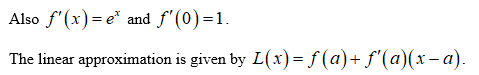# Use a linear approximation (or differentials) to estimate the given number.e−0.01

Question
41 views

Use a linear approximation (or differentials) to estimate the given number.

e−0.01
check_circle

Step 1

Let f(x) = ex, where x = –0.01. Then f(0) = 1.

Step 2Step 3

The linear approximation of the given ...

### Want to see the full answer?

See Solution

#### Want to see this answer and more?

Solutions are written by subject experts who are available 24/7. Questions are typically answered within 1 hour.*

See Solution
*Response times may vary by subject and question.
Tagged in

### Other